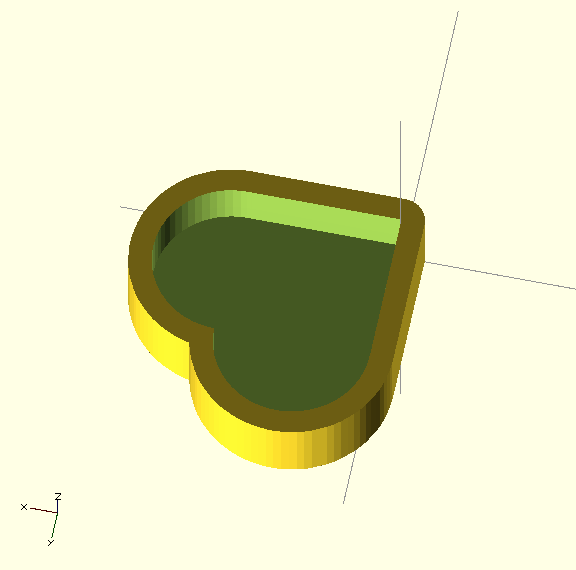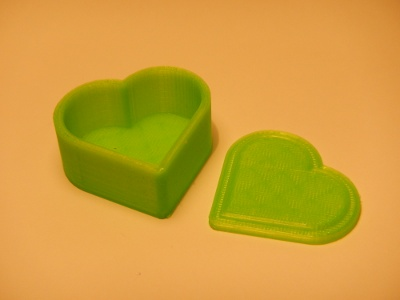Search

18 Jan 2013

## OpenSCAD, the Minkowski sum and a heart-shaped box

In my exploration of openSCAD, the  Minkowski sum looks interesting.  The example given in the Wikibook is of rolling the z-axis edges of a cube:

```minkowski() {
cube(12,center=true);
cylinder(r=4, h=1);
}```although for this task, it's faster to do the minkowski operation in 2-D and then extrude:

```linear_extrude(height=20)
minkowski() {
square(12,center=true);
circle(r=4);
}```

In both cases, the result is a cube of size 20 - the inner cube of size 12 + 2 * 4, the radius of the circle or cylinder

If we want to roll all edges:

```minkowski() {
cube(12,center=true);
cylinder(r=4, h=1);
rotate([90,0,0]) cylinder(r=2, h=1);
rotate([0,90,0]) cylinder(r=2, h=1);
}```

but this takes a minute on my basic laptop to run.

More simply, we can roll all edges by summing with a sphere instead of a cylinder although this takes longer to run:

```minkowski() {
cube(12,center=true);
sphere(r=4);
}```We might also want to put a 45 degree chamfer on the edges. A rough approach could take advantage of the coarse rendering of a sphere:

```\$fn=6;
minkowski() {
cube(12,center=true);
sphere(r=4);
}```

but this is not accurate. A precise approach uses a rotated cube. Here I've defined a module to parameterize the dimensions:

```module chamfered_cube(s,c) {
minkowski() {
cube(s,center=true);
rotate([45,0,0]) cube(c);
rotate([0,45,0]) cube(c);
rotate([0,0,45]) cube(c);
}
}
chamfered_cube(20,4);```The main use I've seen so far for minkoski operator is to create a cookie cutter. Here the operator is used to add a constant width boundary around an arbitrary curve. One of my projects was to make a heart-shaped box, but my first attempts to remove the box inside with a scaled version of the outside yielded odd wall thicknesses. Minkowski is the way to go for irregular shapes.

```module heart_shape(size) {
union() {
square(size,size);
translate([size/2,size,0]) circle(size/2);
translate([size,size/2,0]) circle(size/2);
}
}

module heart(size,thickness,height) {
linear_extrude(height=height)
minkowski() {
heart_shape(size);
circle(thickness+0.1); //because 0 would produce an empty object
}
}

module heart_box(size,height,thickness) {
difference() {
heart(size,thickness,height);
translate([0,0,thickness])  heart(size,0,height);
}
}

heart_box(20,10,3);```The Minkowski sum is used to add a thickness of 3 mm either side of the heart-shaped boundary, giving a wall thickness of 2 * 3. The heart-shape is offset by the thickness for the outside and by zero for the inside of the box, leaving a constant wall thickness of 3.

Making a box and lid, with clearance between box and lid proved a bit trickier. I found the most accurate approach was to start with a heart shape sized to the inside of the lid, requiring 4 shapes with different boundary thicknesses:

• inside lid : 0
• outside lid : thickness + clearance
• inside box : clearance
• outside box : thickness + clearanceThe full code is in Github

I had this printed on a Makerbot and it looks rather good.  The lid is a little sloppy and the depth of the inset is too shallow, so some adjustment is needed.